## Convert date to month name with Text Function in Excel

This post will guide you how to convert date to month name (text string) from an excel date using Text Function in Microsoft Excel. or how to get the month name from a date value in excel.

The Excel TEXT function converts a numeric value into text string with a specified format. You can convert all the standard number formats such as: dates, times, currency to Text string in excel.

The syntax of the TEXT function is as below:

`= TEXT (value, Format code)`

## Convert date to month name with Text Function

If you want to convert the date to a month name, you can use the TEXT function with a specified format code like “mmm”in excel.  You can try to run the following TEXT formula:

`=TEXT(B1,”mmm”)`

Assuming that you want to get the month name from the date value: 12/1/2018 in Cell B1, then you can enter the above TEXT function into Cell C1.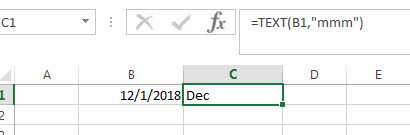If you want to get the full month name, just using the following TEXT function:

`=TEXT(B1,”mmmm”)`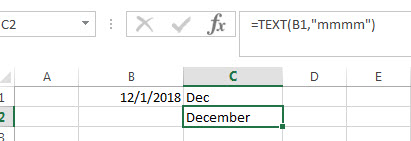In the above formula example, the TEXT formula converts date value to test string using the format code that you specified, based on the format code, only the month name remains in Cell C1 in the last conversion result.

For more format codes in excel formatting, you can refer to the below table:

 Format Code Description Examples 0 only display digits in its place #.00 – Forces the function to display two decimal places =Text(34.234,”\$##.00″) result: \$34.23 # Display the placeholder =Text(4.527,”#.##) result: 4.53 . the position of Decimal Point =Text(342.2,”0.00″) result: 342.20 d Day of the month or day of week d- one or two digit number (1-31) dd- two digit number (01-31) ddd-abbreviated day of week (Mon to Sun) dddd-full name of day of week(Monday to Sunnday) =Text(TODAY(),”DDDD”) result: Monday m The Month of the Year m- one or two digit number mm-two digit number mmm-abbreviated month(Jan to Dec) mmmm-full name of month(January to December)) =Text(TODAY(),”MM/DD/YY”) result:11/06/17 y year yy-two digit representation of year(e.g.01,17) yyyy-four digit representation of year(e.g. 2001,2017) =Text(TODAY(),”MM/DD/YY”) result:11/06/17 h Hour h-one or two digit number (e.g. 1,23) hh-two digit number (e.g. 01,23) =Text(14:16,”hh:mm”) result: 14:16 m Minute m-one or two digit representation (e.g. 1,59) mm-two digit representation (e.g. 01,59) =Text(14:16,”hh:mm”) result: 14:16 s Second s-one or two digit representation (e.g. 1,59) ss=two digit representation (e.g. 01,59)

If you just want to display a month name from 12/1/2018 to “Dec” or “December”in cell B1, you do not need to use a formula, just refer to the following steps:

1# selected the cells you want to change as month format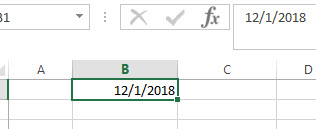2# right click on the selected cells and click on “Format cells…”

3# Select “Custom” under “Number” tab, then type “mmm” or “mmmm” type.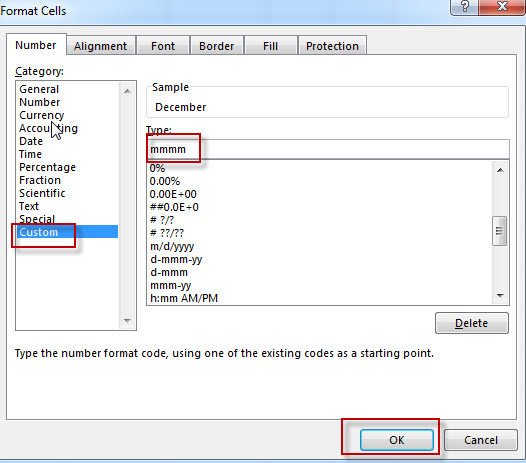4# you will see that the date value is changed to month name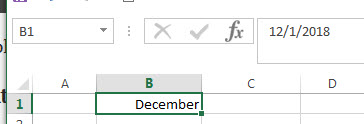### Related Formulas

• Excel Convert numbers to Text
The Text function will accept a numeric value as the first argument, then based on the format code in the second argument to convert the number to text. You can convert all the standard number formats such as: dates, times, currency to Text string in excel.…
• Convert Date to text with Text Function
When you want the dates and Time values as text string, you can use TEXT function in excel to convert dates to text in a specific format code. …
• Convert date to month and year only in excel
If you want to convert the date to month and year only, you can use “yyyymm” format code within the TEXT function in excel, so you can write down the below TEXT formula: =TEXT(date,”yyyymm”)

### Related Functions

• Excel Text function
The Excel TEXT function converts a numeric value into text string with a specified format. The TEXT function is a build-in function in Microsoft Excel and it is categorized as a Text Function. The syntax of the TEXT function is as below: = TEXT (value, Format code)…